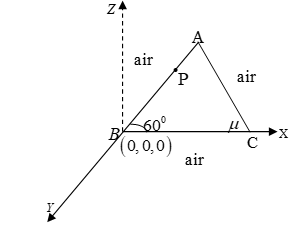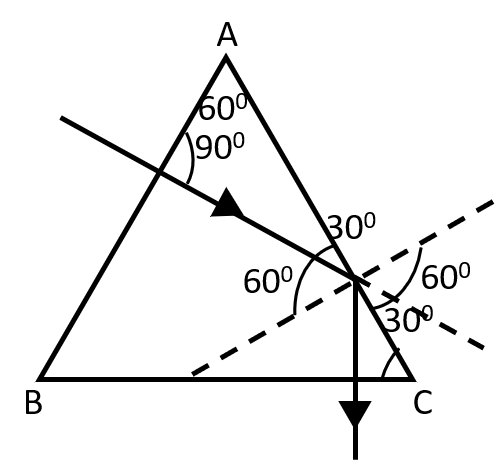Ray optics
Question

# An equilateral prism ABC is placed in air with its base side BC lying horizontally along x-axis. A light ray $\sqrt{3}\mathrm{z}+\mathrm{x}=10$  is incident at a point P on face AB of the prism.If  $\mu$ for the prism is $\frac{3}{2}$ , then net deviation of the ray as it emerges out of the prism is

Difficult
Solution

##For $\mathrm{\mu }=\frac{3}{2},$  total internal reflection takes place at AC. From geometry, it in clear that ray in perpendicular incident on BC, so net deviation = ${180}^{\mathrm{o}}-{120}^{\mathrm{o}}={60}^{\mathrm{o}},$  only at AC.

Get Instant Solutions